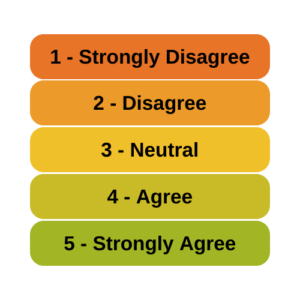# Model Assumptions

### Can Likert Scale Data ever be Continuous?

January 19th, 2023 by

A very common question is whether it is legitimate to use Likert scale data in parametric statistical procedures thatrequire interval data, such as Linear Regression, ANOVA, and Factor Analysis.

A typical Likert scale item has 5 to 11 points that indicate the degree of something. For example, it could measure agreement with a statement, such as 1=Strongly Disagree to 5=Strongly Agree. It can be a 1 to 5 scale, 0 to 10, etc. (more…)

### When to Check Model Assumptions

March 7th, 2016 by

Like the chicken and the egg, there’s a question about which comes first: run a model or test assumptions? Unlike the chickens’, the model’s question has an easy answer.

There are two types of assumptions in a statistical model.  Some are distributional assumptions about the residuals.  Examples include independence, normality, and constant variance in a linear model.

Others are about the form of the model.  They include linearity and (more…)

### The Distribution of Independent Variables in Regression Models

January 19th, 2010 byWhile there are a number of distributional assumptions in regression models, one distribution that has no assumptions is that of any predictor (i.e. independent) variables.

It’s because regression models are directional. In a correlation, there is no direction–Y and X are interchangeable. If you switched them, you’d get the same correlation coefficient.

But regression is inherently a model about the outcome variable. What predicts its value and how well? The nature of how predictors relate to it (more…)

### Checking Assumptions in ANOVA and Linear Regression Models: The Distribution of Dependent Variables

April 10th, 2009 by

Here’s a little reminder for those of you checking assumptions in regression and ANOVA:

The assumptions of normality and homogeneity of variance for linear models are not about Y, the dependent variable.    (If you think I’m either stupid, crazy, or just plain nit-picking, read on.  This distinction really is important). (more…)